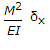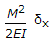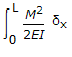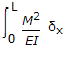# Civil Engineering - Theory of Structures

Exercise : Theory of Structures - Section 1
11.
Stress may be expressed in Newtons
per millimetre square (N/mm2)
per centimetre square (N/cm2)
per metre square (N/m2)
All of the above.
Explanation:
No answer description is available. Let's discuss.

12.
Principal planes are subjected to
normal stresses only
tangential stresses only
normal stresses as well as tangential stresses
none of these.
Explanation:
No answer description is available. Let's discuss.

13.
In plastic analysis, the shape factor for a circular section, is
1.5
1.6
1.7
1.75
Explanation:
No answer description is available. Let's discuss.

14.
Keeping the depth d constant, the width of a cantilever of length l of uniform strength loaded with a uniformly distributed load w varies from zero at the free end and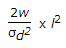at the fixed end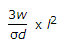at the fixed end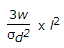at the fixed end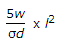at the fixed end